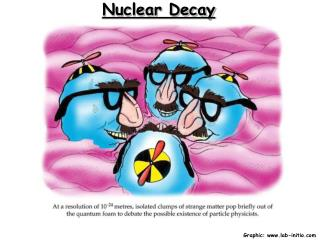DownloadDownload PresentationNuclear Decay

# Nuclear Decay

Télécharger la présentation## Nuclear Decay

- - - - - - - - - - - - - - - - - - - - - - - - - - - E N D - - - - - - - - - - - - - - - - - - - - - - - - - - -
##### Presentation Transcript

1. Nuclear Decay Graphic: www.lab-initio.com

2. Nuclear Symbols Mass number, A (p+ + no) Element symbol Atomic number, Z (number of p+)

3. Balancing Nuclear Equations Areactants = Aproducts 235 + 1 = 142 + 91 + 3(1) 92 + 0 = 56 + 36 + 3(0) Zreactants = Zproducts

4. Balancing Nuclear Equations #2 222 226 = 4 + ____ 222 Rn 86 86 88 = 2 + ___ Atomic number 86 is radon, Rn

5. Balancing Nuclear Equations #3 95 235 + 1 = 139 + 2(1) + ____ 95 Y 39 39 92 + 0 = 53 + 2(0) + ____ Atomic number 39 is yttrium, Y

6. Alpha Decay Alpha production (a): an alpha particle is a helium nucleus Alpha decay is limited to heavy, radioactive nuclei

7. Alpha Radiation Limited to VERY large nucleii.

8. Beta Decay Beta production (b): A beta particle is an electron ejected from the nucleus Beta emission converts a neutron to a proton

9. Beta Radiation Converts a neutron into a proton.

10. Gamma Ray Production Gamma ray production (g): Gamma rays are high energy photons produced in association with other forms of decay. Gamma rays are massless and do not, by themselves, change the nucleus

11. Gamma Ray Production Gamma ray production (g): Gamma rays are high energy photons produced in association with other forms of decay. Gamma rays are massless and do not, by themselves, change the nucleus

12. Positron Production Positron emission: Positrons are the anti-particle of the electron Positron emission converts a proton to a neutron

13. Electron Capture Electron capture: (inner-orbital electron is captured by the nucleus) Electron capture converts a proton to a neutron